# SAT Math Multiple Choice Question 790: Answer and Explanation

### Test Information

Question: 790

10. Anneke is competing in a 500-meter freestyle swim event, which consists of swimmingthe length of a pool 20 times. If Anneke averages 26.4 seconds per length of the pool,which of the following equations could be used to determine the number of meters (m) Anneke has left in the event after swimming for s seconds?

• A.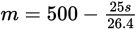• B.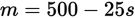• C.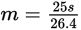• D.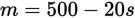Getting to the Answer: You're told that the units of m are meters, so you must find a way to combine the various quantities so that, when simplified, the result will be in meters as well. Anneke will swim 20 lengths in the 500-meter event, which means each length of the pool is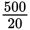= 25 m. Because each length is 25 m and Anneke can swim one length in 26.4 seconds on average, her average speed is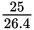m/s. Multiplying this quantity by s, the elapsed race time in seconds, will eliminate seconds from the units. The quantitys can therefore be subtracted from 500 (which is in meters) to yield the number of meters Anneke has remaining, making the final equation m = 500 -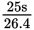. (A) is the correct answer.